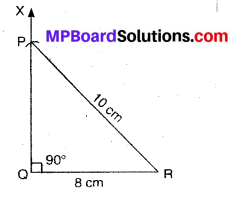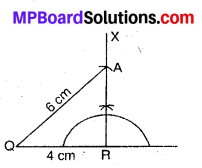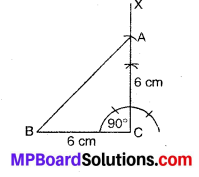# MP Board Class 7th Maths Solutions Chapter 10 Practical Geometry Ex 10.5

## MP Board Class 7th Maths Solutions Chapter 10 Practical Geometry Ex 10.5

Question 1.
Construct the right angled ∆PQR, where m ∠Q = 90°, QR = 8 cm and PR 10 cm.
Solution:
Steps of Construction :1. Draw a line segment QR = 8 cm.
2. Draw ∠RQX = 90°.
3. .With centre R and radius 10 cm (= PR = Hypotenuse), draw an arc to intersect ray QX at P.
4. Join PR.
Then, PQR is the required right angled triangle.Question 2.
Construct a right-angled triangle whose hypotenuse is 6 cm long and one of the legs is 4 cm long.
Solution:
Steps of Construction :1. Draw a line segment QR of length 4 cm.
2. Draw ∠QRX = 90°.
3. With Q as centre and radius 6 cm (= hypotenuse) draw an arc intersecting QX at P.
4. Join PQ.
Then, PQR is the required right-angled triangle.

Question 3.
Construct an isosceles right-angled triangle ABC, where m ∠ACB = 90° and AC = 6 cm.
Solution:
Steps of Construction :1. Draw a line segment BC = 6 cm.
2. At C, draw an angle BCX = 90°
3. From ray CX, cut off CA = 6 cm.
4. Join AB.
Then, ABC is the required an isosceles right-angled triangle.